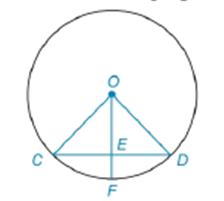Chapter 6.3, Problem 2E### Elementary Geometry for College St...

6th Edition
Daniel C. Alexander + 1 other
ISBN: 9781285195698

#### Solutions

Chapter
Section### Elementary Geometry for College St...

6th Edition
Daniel C. Alexander + 1 other
ISBN: 9781285195698
Textbook Problem
1 views

# Note: Exercises preceded by an asterisk are of a more challenging nature.Given: O C = 8     a n d     O E = 6 O E ¯ ⊥ C D ¯     i n     ⊙ O Find: C DTo determine

To find:

To find CD.

Explanation

Given that OC=8 and OE=6 and OE¯CD¯ in O

Consider the following figure,

Given that OE¯CD¯ in O then ΔOCE is right triangle.

Pythagorean theorem:

In a right angled triangle then the square of the hypotenuse is equal to the sum of the squares of the other two sides.

Consider the ΔOCE to get the following,

OC2=OE2+CE2

Substitute the value of OC=8 and OE=6 to get the following,

### Still sussing out bartleby?

Check out a sample textbook solution.

See a sample solution

#### The Solution to Your Study Problems

Bartleby provides explanations to thousands of textbook problems written by our experts, many with advanced degrees!

Get Started

#### Write each of the following as radicals.

Mathematical Applications for the Management, Life, and Social Sciences

#### Evaluate the integral. 33. 32xx2dx

Single Variable Calculus: Early Transcendentals

#### The implied domain of is: (1, ∞) (−∞, 1) x ≠ 1 (−1, 1)

Study Guide for Stewart's Single Variable Calculus: Early Transcendentals, 8th

#### The quadratic approximation for at a = 1 is:

Study Guide for Stewart's Multivariable Calculus, 8th

#### Identify and describe the steps in the research process.

Research Methods for the Behavioral Sciences (MindTap Course List)